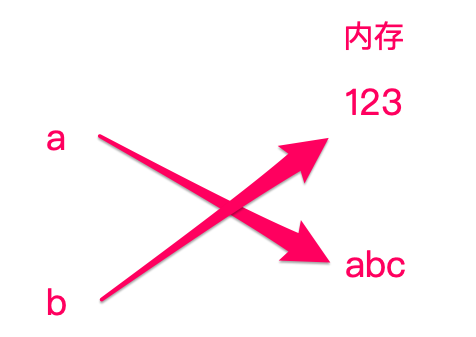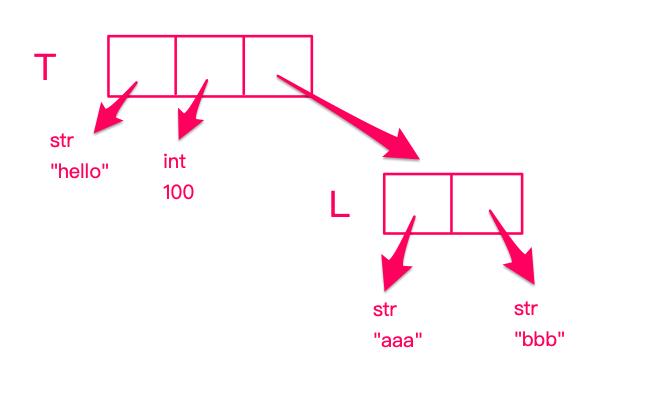# python学习笔记

## 1.注释与缩进

### 1.1 注释

# 开头，一直到行尾。

### 1.2 缩进规则

python中，具有相同缩进的代码被视为代码块。
Python的习惯写法：4个空格，不使用Tab，更不要混合Tab和空格，否则很容易造成因为缩进引起的语法错误。

## 2.数据类型

16 进制 用 0x 作为前缀。

### 2.3 字符串

' '" "''' '''括起来的任意文本。若使用多行文字，用''' '''

print 'hello\nworld'
print '''hello
world'''


hello
world
hello
world

#### 2.3.1 内部使用'和"

print "'hello'"
print '"hello"'

'hello'
"hello"


py print '''"hello" 'world' '''
"hello" 'world'

#### 2.3.2 转义

\和 c/c++ 的用法一样，可使用\n等。
r'...'是将''内部分一串都转义，但是不能在内部包含'".

print r'\\\hhh'


\\hhh

print r'''he said, "hi".'''


he said, "hi".

#### 2.3.3 字符串拼接

py print 'hello' + ' ' + 'world'

hello world

（不可将字符串与其他类型用 + 连接）

py print 'hello', 'world'

hello world

#### 2.3.4 中文字符

# -*- coding: utf-8 -*-


### 2.4 布尔值

#### 2.4.1 基本概念

TrueFalse（首字母大写）。

Python把0、空字符串''None看成 False，其他数值和非空字符串都看成 True。

#### 2.4.2 短路运算

1. 在计算 a and b 时，如果 a 是 False，则根据与运算法则，整个结果必定为 False，因此返回 a；如果 a 是 True，则整个计算结果必定取决与 b，因此返回 b。

2. 在计算 a or b 时，如果 a 是 True，则根据或运算法则，整个计算结果必定为 True，因此返回 a；如果 a 是 False，则整个计算结果必定取决于 b，因此返回 b。

a = True
print a and 'a=T' or 'a=F'


a=T

### 2.5 空值

None 表示，并不是 0，因为 0 是有意义的。

## 3.变量

### 3.2 动态语言

python的变量本身类型不固定，因此称 python 为动态语言。

a = 123
print a
a = 'hello world'
print a


123
hello world

### 3.2 具体分析

a = 123
b = a
a = 'abc'
print b


123## 4.list / tuple 类型

### 4.1 list

#### 4.1.1 构造

list（列表） 是 python 的内置类型，使用方法也很简单。

L = ['hello', 'and', 'python']
print L


['hello', 'and', 'python']

list 中的元素类型也不要求一样。

L = ['hello', 100, 'python']
print L


['hello', 100, 'python']

empty_list = []


#### 4.1.2 使用

L = ['hello', 100, 'python']
print L
print L
print L


hello
100
python

list 还可以倒序输出:

L = ['hello', 100, 'python']
print L[-1]
print L[-2]
print L[-3]


python
100
hello

#### 4.1.3 添加新元素

L = ['hello', 100, 'python']
L.append(200)
print L
L.insert(1, 'world')
print L

['hello', 100, 'python', 200]
['hello', 'world', 100, 'python', 200]


#### 4.1.4 删除元素

L = ['hello', 100, 'python', 'hehe', 200]
L.pop()
print L
L.pop(2)
print L

['hello', 100, 'python', 'hehe']
['hello', 100, 'hehe']


#### 4.1.5 替换元素

L = ['hello', 100, 'python', 'hehe', 200]
L = 'sss'
print L
L[-2] = 'haha'
print L

['hello', 100, 'sss', 'hehe', 200]
['hello', 100, 'sss', 'haha', 200]


### 4.2 tuple

#### 4.2.1 构造

tuple（元组）与 list 非常类似，但 tuple 一旦创建完，就不能修改。
tuple 用()来括起来：

T = ('hello', 100, 'python', 'hehe', 200)
print T


#### 4.2.2 单元素的tuple

python 规定若 tuple 只有一个元素时，需要在第一个元素后面加上逗号：

T = ('hello', )
print T


(hello)

#### 4.2.3 可变tuple

T = ('hello', 100, ['aaa', 'bbb'])
L = T
L = 'xxx'
print T


('hello', 100, ['xxx', 'bbb'])## 5.条件判断与循环

### 5.1 if

if 10 > 9:
print 'yes'


yes

if 后面还可加 not：

if not 9 > 10:
print 'no'


no

: 后面，代表代码块的开始。

### 5.2 if-else

if 11 > 10:
print 'yes'
else:
print 'no'


yes

### 5.3 if-elif-else

age = 3
if age >= 18:
elif age >= 6:
print 'teenager'
elif age >= 3:
print 'kid'
else:
print 'baby'


kid

### 5.4 for循环

L = ['hello', 'why', 'yes']
for str in L:
print str


hello
why
yes

str 变量是在 for 循环中定义的，意思是依次取出 list 中的每一个元素，赋值给 str ，然后执行缩进中的内容。

### 5.5 while循环

a = 1
while a < 5:
print a
a += 1


1
2
3
4

### 5.6 break与continue

#### 5.6.1 break

a = 1
while True:
print a
a += 1
if a > 5:
break


## 6 dict 和 set 类型

### 6.1 dict

#### 6.1.1 构造

d = {
'Amon': 90,
'Kiki': 95,
'Jack': 80
}
print d
print len(d)


{'Kiki': 95, 'Amon': 90, 'Jack': 80}
3

#### 6.1.2 使用

d[key] = value，如下：

d = {
'Amon': 90,
'Kiki': 95,
'Jack': 80
}
print d['Amon']


d = {
'Amon': 90,
'Kiki': 95,
'Jack': 80
}
print d['Tina']


Traceback (most recent call last):
File "hello.py", line 6, in <module>
print d['Tina']
KeyError: 'Tina'


if 'Tina' in d:
print d['Tina']


print d.get('Tina')


#### 6.1.3 dict的特点

1.key 不能重复，且 key 不能变（所以 list 不能作为key）
2.在 dict 中，元素是没有顺序的（所以不能用d, d...)
3.查找速度快，但占用内存大

#### 6.1.4 更新dict

d = {
'Amon': 90,
'Kiki': 95,
'Jack': 80
}
d['newPerson'] = 60
print d


{'newPerson': 60, 'Kiki': 95, 'Amon': 90, 'Jack': 80}

#### 6.1.5 遍历dict

d = {
'Amon': 90,
'Kiki': 95,
'Jack': 80
}
for key in d:
print key, d[key]


Kiki 95
Amon 90
Jack 80

### 6.2 set

#### 6.2.1 构造

set 与 dict 的区别的，dict 中存的是一个 key-value 的键值对，而 set 中只有 key。
set 中的 key 也不能重复，相当于 set 是一个不能有重复元素的集合，且元素无序。
set 的调用方法是调用他的构造函数 set()，在里面传入一个 list，list 中存的是需要传入的 key，用法如下：

s = set(['Amon', 'Kiki', 'Tina'])
print s


set(['Kiki', 'Amon', 'Tina'])

#### 6.2.2 使用

s = set(['Amon', 'Kiki', 'Tina'])
print 'kkk' in s


False

#### 6.2.3 set 的特点

1.元素不能重复，且不可变（所以 list 不能作为 set 的元素）
2.在 set 中，元素没有顺序（所以不能用 s，s...）
3.判断某个元素是否在 set 中的速度很快

#### 6.2.4 遍历 set

s = set(['Amon', 'Kiki', 'Tina'])
for name in s:
print name


Kiki
Amon
Tina

#### 6.2.5 更新 set

（若删除元素不存在，会报错）

s = set(['Amon', 'Kiki', 'Tina'])
print s
s.remove('Amon')
print s


set([100, 'Kiki', 'Amon', 'Tina'])
set([100, 'Kiki', 'Tina'])

## 7.函数

### 7.1 python内置函数

python 的官方文档：
http://docs.python.org/2/library/functions.html#abs

print help(abs)

Help on built-in function abs in module __builtin__:

abs(...)
abs(number) -> number

Return the absolute value of the argument.


### 7.2 编写函数

def my_abs(x):
if x >= 0:
return x
else:
return -x
a = -3
print my_abs(a)


3

### 7.3 返回多值

def my_abs(x1, x2, x3):
return abs(x1), abs(x2), abs(x3)
a = -3
b = 2
c = -5
print my_abs(a, b, c)


(3, 2, 5)

### 7.4 默认参数

def sum(x1, x2, x3 = 0, x4 = 0):
return x1 + x2 + x3 + x4
a = -3
b = 2
print sum(a, b)


-1

### 7.5 可变参数

def sum(*args):
ans = 0
for em in args:
ans += em
return ans
print sum(2, 3, -6)


-1

## 8.切片

### 8.1 切片简述

L = [4, 26, 8, 2, 11, 33, 27]
for i in range(5):
print L[i]


4
26
8
2
11

range(n) 代表 0~（n-1）之间的数，让 i 等于这些数，再调用 L[i]。

L = [4, 26, 8, 2, 11, 33, 27]
print L[0:5]


[4, 26, 8, 2, 11]

[a, b] 是一个前闭后开的区间，代表第 a 个元素到第 b 个元素，但不包括第 b 个。
[:]代表所有元素。
[::a]代表每 a 个元素取一个元素。

L = [4, 26, 8, 2, 11, 33, 27]
print L[::2]


[4, 8, 11, 27]

### 8.2 倒序切片

python 支持 L[-1] 取最后一个元素，同样也支持 L[-1:] 倒数切片：

L = [4, 26, 8, 2, 11, 33, 27]
print L[-3:]
print L[:-3]
print L[-5:-2]
print L[-6:-2:2]


[11, 33, 27] （倒数三个数）
[4, 26, 8, 2] （第一个数到倒数第三个数（不包括））
[8, 2, 11]（倒数第五个数到倒数第三个数（不包括））
[26, 2]（每2个中1个，倒数第六个数到倒数第二个数（不包括））

### 8.3 字符串切片

print 'hello world'[2:7]


llo w

## 9.迭代

### 9.2 索引迭代

Python中，迭代永远是取出元素本身，而非元素的索引（下标）。

L = ['Amon', 'Kiki', 'Tina']
=> L = [(0, 'Amon'), (1, 'Kiki'), (2, 'Tina')]

L = ['Amon', 'Kiki', 'Tina']
for i, name in enumerate(L):
print i, name


0 Amon
1 Kiki
2 Tina

### 9.3 迭代dict

dict 在 for 循环中，每次可以取出他的一个 key。若我们想迭代 value ，可用 values() 方法。

d = {
'Amon': 90,
'Tina': 60,
'Kiki': 80
}
print d.values()


[80, 90, 60]
80
90
60

values() 方法实际上将 dict 转化为一个包含 values 的 list。

d = {
'Amon': 90,
'Tina': 60,
'Kiki': 80
}
print d.itervalues()


<dictionary-valueiterator object at 0x102930730>
80
90
60

itervalues() 方法不会将 dict 转换，而是在每次迭代过程中，取出 value，所以更节约内存。

d = {
'Amon': 90,
'Tina': 60,
'Kiki': 80
}
print d.items()


[('Kiki', 80), ('Amon', 90), ('Tina', 60)]

d = {
'Amon': 90,
'Tina': 60,
'Kiki': 80
}
for key, value in d.items():
print key, ':', value


Kiki : 80
Amon : 90
Tina : 60

## 10.列表生成式

### 10.1 简述

L = [x * x for x in range(1, 11)]
print L


[1, 4, 9, 16, 25, 36, 49, 64, 81, 100]

L = [x * x for x in range(1, 11) if (x % 2 == 0)]
print L


[4, 16, 36, 64, 100]

### 10.2 多层嵌套

print [a + str(b) for a in 'avsde' for b in range(1, 3)]


['a1', 'a2', 'v1', 'v2', 's1', 's2', 'd1', 'd2', 'e1', 'e2']

## 参考

https://www.imooc.com/learn/177